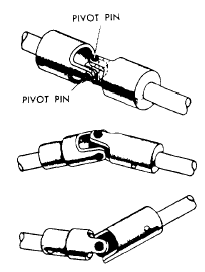Google+ 機動學論壇（TALKING MECHANISMS）: Universal Joint 萬向接頭

## 2007年6月16日 星期六

### Universal Joint 萬向接頭

5

B94611012 黃振瑋

Robert Hooke 製造了實用的接頭並為他命名為"Hooke's joint"，而"Universal Joint"這個名稱則是美國的汽車大亨 Ford 所給的!

http://en.wikipedia.org/wiki/Image:Universal_joint.gif$homokinetic 聯接$$擴大$

function [th2, omega2, alpha2]=unijoint(betax,theta3,omega3)

betax：兩軸間之夾角。
theta3：迴轉之角度。

function [th2, omega2, alpha2]=unijoint(betax,theta3,omega3)
%
% [th2, omega2, alpha2]=unijoint(betax,theta3,omega3)
% This program calculate the displacement, velocity and acceleration of a universal joint
% Inputs:
% betax: angle between two axes, degrees
% theta3: angle of rotations, degrees
% omega3: angular velocity, rad/sec
% Example:[t,v,a]=unijoint(45,0:10:360,1)
%
d2g=pi/180;
axang=betax*d2g;
th3=theta3*d2g;
cb=cos(axang);sb=sin(axang);
st=sin(th3);ct=cos(th3);
th2=atan(tan(th3)*cb);
omega2=cb./(1-(st.*sb).^2).*omega3;
alpha2=(omega3.*omega3.*cb.*sb.*sb).*sin(2*th3)./(1-(st.*sb).^2).^2;